# sklearn.cluster.MiniBatchKMeans¶

class sklearn.cluster.MiniBatchKMeans(n_clusters=8, *, init='k-means++', max_iter=100, batch_size=1024, verbose=0, compute_labels=True, random_state=None, tol=0.0, max_no_improvement=10, init_size=None, n_init='warn', reassignment_ratio=0.01)[source]

Mini-Batch K-Means clustering.

Read more in the User Guide.

Parameters:
n_clustersint, default=8

The number of clusters to form as well as the number of centroids to generate.

init{‘k-means++’, ‘random’}, callable or array-like of shape (n_clusters, n_features), default=’k-means++’

Method for initialization:

‘k-means++’ : selects initial cluster centroids using sampling based on an empirical probability distribution of the points’ contribution to the overall inertia. This technique speeds up convergence. The algorithm implemented is “greedy k-means++”. It differs from the vanilla k-means++ by making several trials at each sampling step and choosing the best centroid among them.

‘random’: choose n_clusters observations (rows) at random from data for the initial centroids.

If an array is passed, it should be of shape (n_clusters, n_features) and gives the initial centers.

If a callable is passed, it should take arguments X, n_clusters and a random state and return an initialization.

max_iterint, default=100

Maximum number of iterations over the complete dataset before stopping independently of any early stopping criterion heuristics.

batch_sizeint, default=1024

Size of the mini batches. For faster computations, you can set the batch_size greater than 256 * number of cores to enable parallelism on all cores.

Changed in version 1.0: batch_size default changed from 100 to 1024.

verboseint, default=0

Verbosity mode.

compute_labelsbool, default=True

Compute label assignment and inertia for the complete dataset once the minibatch optimization has converged in fit.

random_stateint, RandomState instance or None, default=None

Determines random number generation for centroid initialization and random reassignment. Use an int to make the randomness deterministic. See Glossary.

tolfloat, default=0.0

Control early stopping based on the relative center changes as measured by a smoothed, variance-normalized of the mean center squared position changes. This early stopping heuristics is closer to the one used for the batch variant of the algorithms but induces a slight computational and memory overhead over the inertia heuristic.

To disable convergence detection based on normalized center change, set tol to 0.0 (default).

max_no_improvementint, default=10

Control early stopping based on the consecutive number of mini batches that does not yield an improvement on the smoothed inertia.

To disable convergence detection based on inertia, set max_no_improvement to None.

init_sizeint, default=None

Number of samples to randomly sample for speeding up the initialization (sometimes at the expense of accuracy): the only algorithm is initialized by running a batch KMeans on a random subset of the data. This needs to be larger than n_clusters.

If None, the heuristic is init_size = 3 * batch_size if 3 * batch_size < n_clusters, else init_size = 3 * n_clusters.

n_init‘auto’ or int, default=3

Number of random initializations that are tried. In contrast to KMeans, the algorithm is only run once, using the best of the n_init initializations as measured by inertia. Several runs are recommended for sparse high-dimensional problems (see Clustering sparse data with k-means).

When n_init='auto', the number of runs depends on the value of init: 3 if using init='random' or init is a callable; 1 if using init='k-means++' or init is an array-like.

New in version 1.2: Added ‘auto’ option for n_init.

Changed in version 1.4: Default value for n_init will change from 3 to 'auto' in version 1.4.

reassignment_ratiofloat, default=0.01

Control the fraction of the maximum number of counts for a center to be reassigned. A higher value means that low count centers are more easily reassigned, which means that the model will take longer to converge, but should converge in a better clustering. However, too high a value may cause convergence issues, especially with a small batch size.

Attributes:
cluster_centers_ndarray of shape (n_clusters, n_features)

Coordinates of cluster centers.

labels_ndarray of shape (n_samples,)

Labels of each point (if compute_labels is set to True).

inertia_float

The value of the inertia criterion associated with the chosen partition if compute_labels is set to True. If compute_labels is set to False, it’s an approximation of the inertia based on an exponentially weighted average of the batch inertiae. The inertia is defined as the sum of square distances of samples to their cluster center, weighted by the sample weights if provided.

n_iter_int

Number of iterations over the full dataset.

n_steps_int

Number of minibatches processed.

New in version 1.0.

n_features_in_int

Number of features seen during fit.

New in version 0.24.

feature_names_in_ndarray of shape (n_features_in_,)

Names of features seen during fit. Defined only when X has feature names that are all strings.

New in version 1.0.

KMeans

The classic implementation of the clustering method based on the Lloyd’s algorithm. It consumes the whole set of input data at each iteration.

Notes

When there are too few points in the dataset, some centers may be duplicated, which means that a proper clustering in terms of the number of requesting clusters and the number of returned clusters will not always match. One solution is to set reassignment_ratio=0, which prevents reassignments of clusters that are too small.

Examples

>>> from sklearn.cluster import MiniBatchKMeans
>>> import numpy as np
>>> X = np.array([[1, 2], [1, 4], [1, 0],
...               [4, 2], [4, 0], [4, 4],
...               [4, 5], [0, 1], [2, 2],
...               [3, 2], [5, 5], [1, -1]])
>>> # manually fit on batches
>>> kmeans = MiniBatchKMeans(n_clusters=2,
...                          random_state=0,
...                          batch_size=6,
...                          n_init="auto")
>>> kmeans = kmeans.partial_fit(X[0:6,:])
>>> kmeans = kmeans.partial_fit(X[6:12,:])
>>> kmeans.cluster_centers_
array([[3.375, 3.  ],
[0.75 , 0.5 ]])
>>> kmeans.predict([[0, 0], [4, 4]])
array([1, 0], dtype=int32)
>>> # fit on the whole data
>>> kmeans = MiniBatchKMeans(n_clusters=2,
...                          random_state=0,
...                          batch_size=6,
...                          max_iter=10,
...                          n_init="auto").fit(X)
>>> kmeans.cluster_centers_
array([[3.55102041, 2.48979592],
[1.06896552, 1.        ]])
>>> kmeans.predict([[0, 0], [4, 4]])
array([1, 0], dtype=int32)


Methods

 fit(X[, y, sample_weight]) Compute the centroids on X by chunking it into mini-batches. fit_predict(X[, y, sample_weight]) Compute cluster centers and predict cluster index for each sample. fit_transform(X[, y, sample_weight]) Compute clustering and transform X to cluster-distance space. get_feature_names_out([input_features]) Get output feature names for transformation. Get metadata routing of this object. get_params([deep]) Get parameters for this estimator. partial_fit(X[, y, sample_weight]) Update k means estimate on a single mini-batch X. predict(X[, sample_weight]) Predict the closest cluster each sample in X belongs to. score(X[, y, sample_weight]) Opposite of the value of X on the K-means objective. set_fit_request(*[, sample_weight]) Request metadata passed to the fit method. set_output(*[, transform]) Set output container. set_params(**params) Set the parameters of this estimator. set_partial_fit_request(*[, sample_weight]) Request metadata passed to the partial_fit method. set_predict_request(*[, sample_weight]) Request metadata passed to the predict method. set_score_request(*[, sample_weight]) Request metadata passed to the score method. Transform X to a cluster-distance space.
fit(X, y=None, sample_weight=None)[source]

Compute the centroids on X by chunking it into mini-batches.

Parameters:
X{array-like, sparse matrix} of shape (n_samples, n_features)

Training instances to cluster. It must be noted that the data will be converted to C ordering, which will cause a memory copy if the given data is not C-contiguous. If a sparse matrix is passed, a copy will be made if it’s not in CSR format.

yIgnored

Not used, present here for API consistency by convention.

sample_weightarray-like of shape (n_samples,), default=None

The weights for each observation in X. If None, all observations are assigned equal weight. sample_weight is not used during initialization if init is a callable or a user provided array.

New in version 0.20.

Returns:
selfobject

Fitted estimator.

fit_predict(X, y=None, sample_weight=None)[source]

Compute cluster centers and predict cluster index for each sample.

Convenience method; equivalent to calling fit(X) followed by predict(X).

Parameters:
X{array-like, sparse matrix} of shape (n_samples, n_features)

New data to transform.

yIgnored

Not used, present here for API consistency by convention.

sample_weightarray-like of shape (n_samples,), default=None

The weights for each observation in X. If None, all observations are assigned equal weight.

Returns:
labelsndarray of shape (n_samples,)

Index of the cluster each sample belongs to.

fit_transform(X, y=None, sample_weight=None)[source]

Compute clustering and transform X to cluster-distance space.

Equivalent to fit(X).transform(X), but more efficiently implemented.

Parameters:
X{array-like, sparse matrix} of shape (n_samples, n_features)

New data to transform.

yIgnored

Not used, present here for API consistency by convention.

sample_weightarray-like of shape (n_samples,), default=None

The weights for each observation in X. If None, all observations are assigned equal weight.

Returns:
X_newndarray of shape (n_samples, n_clusters)

X transformed in the new space.

get_feature_names_out(input_features=None)[source]

Get output feature names for transformation.

The feature names out will prefixed by the lowercased class name. For example, if the transformer outputs 3 features, then the feature names out are: ["class_name0", "class_name1", "class_name2"].

Parameters:
input_featuresarray-like of str or None, default=None

Only used to validate feature names with the names seen in fit.

Returns:
feature_names_outndarray of str objects

Transformed feature names.

Get metadata routing of this object.

Please check User Guide on how the routing mechanism works.

Returns:

A MetadataRequest encapsulating routing information.

get_params(deep=True)[source]

Get parameters for this estimator.

Parameters:
deepbool, default=True

If True, will return the parameters for this estimator and contained subobjects that are estimators.

Returns:
paramsdict

Parameter names mapped to their values.

partial_fit(X, y=None, sample_weight=None)[source]

Update k means estimate on a single mini-batch X.

Parameters:
X{array-like, sparse matrix} of shape (n_samples, n_features)

Training instances to cluster. It must be noted that the data will be converted to C ordering, which will cause a memory copy if the given data is not C-contiguous. If a sparse matrix is passed, a copy will be made if it’s not in CSR format.

yIgnored

Not used, present here for API consistency by convention.

sample_weightarray-like of shape (n_samples,), default=None

The weights for each observation in X. If None, all observations are assigned equal weight. sample_weight is not used during initialization if init is a callable or a user provided array.

Returns:
selfobject

Return updated estimator.

predict(X, sample_weight='deprecated')[source]

Predict the closest cluster each sample in X belongs to.

In the vector quantization literature, cluster_centers_ is called the code book and each value returned by predict is the index of the closest code in the code book.

Parameters:
X{array-like, sparse matrix} of shape (n_samples, n_features)

New data to predict.

sample_weightarray-like of shape (n_samples,), default=None

The weights for each observation in X. If None, all observations are assigned equal weight.

Deprecated since version 1.3: The parameter sample_weight is deprecated in version 1.3 and will be removed in 1.5.

Returns:
labelsndarray of shape (n_samples,)

Index of the cluster each sample belongs to.

score(X, y=None, sample_weight=None)[source]

Opposite of the value of X on the K-means objective.

Parameters:
X{array-like, sparse matrix} of shape (n_samples, n_features)

New data.

yIgnored

Not used, present here for API consistency by convention.

sample_weightarray-like of shape (n_samples,), default=None

The weights for each observation in X. If None, all observations are assigned equal weight.

Returns:
scorefloat

Opposite of the value of X on the K-means objective.

set_fit_request(*, sample_weight: Union[bool, None, str] = '$UNCHANGED$') [source]

Request metadata passed to the fit method.

Note that this method is only relevant if enable_metadata_routing=True (see sklearn.set_config). Please see User Guide on how the routing mechanism works.

The options for each parameter are:

• True: metadata is requested, and passed to fit if provided. The request is ignored if metadata is not provided.

• False: metadata is not requested and the meta-estimator will not pass it to fit.

• None: metadata is not requested, and the meta-estimator will raise an error if the user provides it.

• str: metadata should be passed to the meta-estimator with this given alias instead of the original name.

The default (sklearn.utils.metadata_routing.UNCHANGED) retains the existing request. This allows you to change the request for some parameters and not others.

New in version 1.3.

Note

This method is only relevant if this estimator is used as a sub-estimator of a meta-estimator, e.g. used inside a Pipeline. Otherwise it has no effect.

Parameters:
sample_weightstr, True, False, or None, default=sklearn.utils.metadata_routing.UNCHANGED

Metadata routing for sample_weight parameter in fit.

Returns:
selfobject

The updated object.

set_output(*, transform=None)[source]

Set output container.

See Introducing the set_output API for an example on how to use the API.

Parameters:
transform{“default”, “pandas”}, default=None

Configure output of transform and fit_transform.

• "default": Default output format of a transformer

• "pandas": DataFrame output

• None: Transform configuration is unchanged

Returns:
selfestimator instance

Estimator instance.

set_params(**params)[source]

Set the parameters of this estimator.

The method works on simple estimators as well as on nested objects (such as Pipeline). The latter have parameters of the form <component>__<parameter> so that it’s possible to update each component of a nested object.

Parameters:
**paramsdict

Estimator parameters.

Returns:
selfestimator instance

Estimator instance.

set_partial_fit_request(*, sample_weight: Union[bool, None, str] = '$UNCHANGED$') [source]

Request metadata passed to the partial_fit method.

Note that this method is only relevant if enable_metadata_routing=True (see sklearn.set_config). Please see User Guide on how the routing mechanism works.

The options for each parameter are:

• True: metadata is requested, and passed to partial_fit if provided. The request is ignored if metadata is not provided.

• False: metadata is not requested and the meta-estimator will not pass it to partial_fit.

• None: metadata is not requested, and the meta-estimator will raise an error if the user provides it.

• str: metadata should be passed to the meta-estimator with this given alias instead of the original name.

The default (sklearn.utils.metadata_routing.UNCHANGED) retains the existing request. This allows you to change the request for some parameters and not others.

New in version 1.3.

Note

This method is only relevant if this estimator is used as a sub-estimator of a meta-estimator, e.g. used inside a Pipeline. Otherwise it has no effect.

Parameters:
sample_weightstr, True, False, or None, default=sklearn.utils.metadata_routing.UNCHANGED

Metadata routing for sample_weight parameter in partial_fit.

Returns:
selfobject

The updated object.

set_predict_request(*, sample_weight: Union[bool, None, str] = '$UNCHANGED$') [source]

Request metadata passed to the predict method.

Note that this method is only relevant if enable_metadata_routing=True (see sklearn.set_config). Please see User Guide on how the routing mechanism works.

The options for each parameter are:

• True: metadata is requested, and passed to predict if provided. The request is ignored if metadata is not provided.

• False: metadata is not requested and the meta-estimator will not pass it to predict.

• None: metadata is not requested, and the meta-estimator will raise an error if the user provides it.

• str: metadata should be passed to the meta-estimator with this given alias instead of the original name.

The default (sklearn.utils.metadata_routing.UNCHANGED) retains the existing request. This allows you to change the request for some parameters and not others.

New in version 1.3.

Note

This method is only relevant if this estimator is used as a sub-estimator of a meta-estimator, e.g. used inside a Pipeline. Otherwise it has no effect.

Parameters:
sample_weightstr, True, False, or None, default=sklearn.utils.metadata_routing.UNCHANGED

Metadata routing for sample_weight parameter in predict.

Returns:
selfobject

The updated object.

set_score_request(*, sample_weight: Union[bool, None, str] = '$UNCHANGED$') [source]

Request metadata passed to the score method.

Note that this method is only relevant if enable_metadata_routing=True (see sklearn.set_config). Please see User Guide on how the routing mechanism works.

The options for each parameter are:

• True: metadata is requested, and passed to score if provided. The request is ignored if metadata is not provided.

• False: metadata is not requested and the meta-estimator will not pass it to score.

• None: metadata is not requested, and the meta-estimator will raise an error if the user provides it.

• str: metadata should be passed to the meta-estimator with this given alias instead of the original name.

The default (sklearn.utils.metadata_routing.UNCHANGED) retains the existing request. This allows you to change the request for some parameters and not others.

New in version 1.3.

Note

This method is only relevant if this estimator is used as a sub-estimator of a meta-estimator, e.g. used inside a Pipeline. Otherwise it has no effect.

Parameters:
sample_weightstr, True, False, or None, default=sklearn.utils.metadata_routing.UNCHANGED

Metadata routing for sample_weight parameter in score.

Returns:
selfobject

The updated object.

transform(X)[source]

Transform X to a cluster-distance space.

In the new space, each dimension is the distance to the cluster centers. Note that even if X is sparse, the array returned by transform will typically be dense.

Parameters:
X{array-like, sparse matrix} of shape (n_samples, n_features)

New data to transform.

Returns:
X_newndarray of shape (n_samples, n_clusters)

X transformed in the new space.

## Examples using sklearn.cluster.MiniBatchKMeans¶Biclustering documents with the Spectral Co-clustering algorithm

Biclustering documents with the Spectral Co-clustering algorithm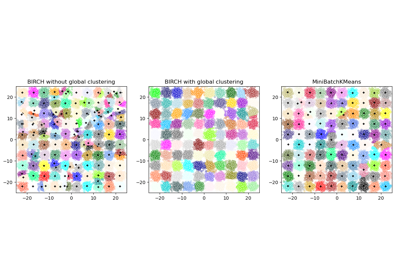Compare BIRCH and MiniBatchKMeans

Compare BIRCH and MiniBatchKMeans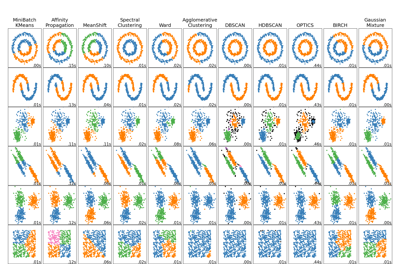Comparing different clustering algorithms on toy datasets

Comparing different clustering algorithms on toy datasets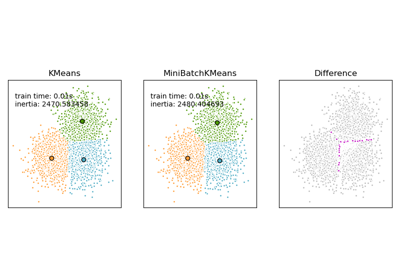Comparison of the K-Means and MiniBatchKMeans clustering algorithms

Comparison of the K-Means and MiniBatchKMeans clustering algorithms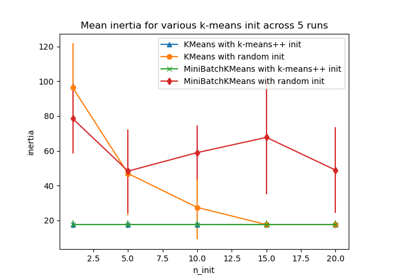Empirical evaluation of the impact of k-means initialization

Empirical evaluation of the impact of k-means initialization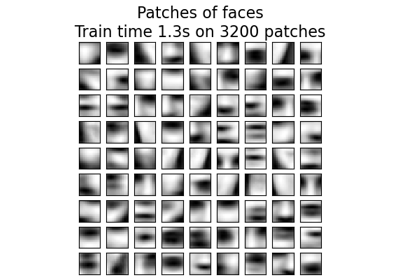Online learning of a dictionary of parts of faces

Online learning of a dictionary of parts of faces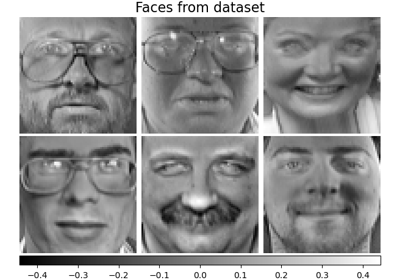Faces dataset decompositions

Faces dataset decompositions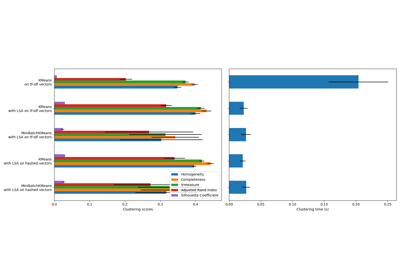Clustering text documents using k-means

Clustering text documents using k-means